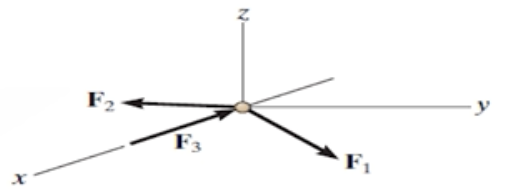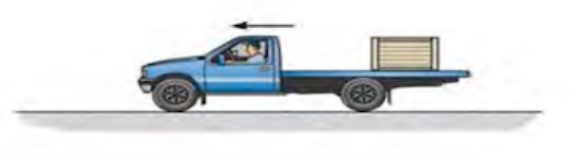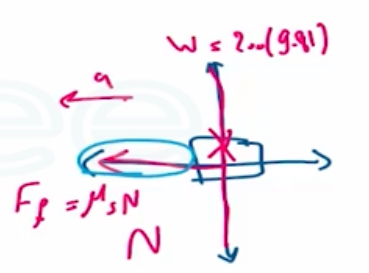Need Help?

Subscribe to Dynamic

###### \${selected_topic_name}
• Notes
• Comments & Questions

$\begin{array}{l}{1.3-1 . \text { The } 6-1 b \text { particle is subjected to the action of its }} \\ {\text { weight and forces } \mathbf{F}_{1}=\{2 \mathbf{i}+6 \mathbf{j}-2 t \mathbf{k}\} \text { lb, } \mathbf{F}_{2}=} \\ {\left\{t^{2} \mathbf{i}-4 t \mathbf{j}-1 \mathbf{k}\right\} \text { lb, and } \mathbf{F}_{3}=\{-2 t \mathbf{i}\} \text { Ib, where } t \text { is in }} \\ {\text { seconds. Determine the distance the ball is from the origin }} \\ {2 \mathrm{s} \text { after being released from rest. }}\end{array}$$w=6l b$

${F_{1}=\left(2 i+6 j-2 t k\right)lb}$

$F_{2}=\left(t^{2}i-4 t j-1 k\right) lb$

$F_{3}=(-2 t i) l b$

$t=2s$

$d V=a d t$

$ds=vdt$

@ $t=2S$

$\Sigma F=m{a}$

${\Sigma F=F_{1}+F_{2}+F_{3}+w}\$

$∴(2 i+6 j-2tk)+\left(t^{2} i-4 t j-1k\right)-2 t i-6 k=\left(\frac{w}{g}\right)(a_xi+a_yj+a_z k)$

$\left(t^{2}-2 t+2\right) i+(-4t+6) j+(-2 t-7) k=\frac{6}{32.2}\left(a_{x}i+a_y j+a_{z} k\right)$

$\frac{6}{32.2} a_{x}=t^{2-} 2 t+2$

$\frac{6}{32.2}v_x=\frac{t^{3}}{3}-\frac{2 t^{2}}{2}+2 t$

${\frac{6}{32.2}} S_{x}=\frac{t^{4}}{12}-\frac{t^{3}}{3}+t^{2}$

$S_{x}=14.31 \mathrm{ft}$

$\frac{6}{32.2} a_ y=-4 t+6$

$\frac{6}{32.2} v_y=-2t^{^{2}}+6 t\$

$\frac{6}{32.2} s_y= \frac{-2 t^{3}}{3}+3 t^{2}$

$s_y=35.78f t$

$\frac{6}{32.2}a_ z=-2 t-7$

$\frac{6}{32.2} v_{z}=-t^{2}-7 t$

$\frac{6}{32.2} S_{z}= \frac{-t^{3}}{3}-\frac{7 t^{2}}{2}$

$s_ z=-89.44 ft$

$S={s_ x^{2}+s_ y^{2}+s_ z^{2}}=97.4 \mathrm{ft}$

$\begin{array}{l}{13-35 . \text { The coefficient of static friction between the } 200-\mathrm{kg}} \\ {\text { crate and the flat bed of the truck is } \mu_{s}=0.3 \text { . Determine }} \\ {\text { the shortest time for the truck to reach a speed of } 60 \mathrm{km} / \mathrm{h} \text { , }} \\ {\text { starting from rest with constant acceleration, so that the }} \\ {\text { crate does not slip. }}\end{array}$$m=2 0 0 k g$

$\mu_{s}=0.3$

$v=60 \mathrm{km} / \mathrm{h}$

$V_{0}=0$

$a=cons t.$

$+ \uparrow{\Sigma Fy}=may$

$∴ N-200(9.81)=0 \Rightarrow N=1962 \mathrm{N}$

$\stackrel{+}{\rightarrow}\ \Sigma F{x}=m a{x} \Rightarrow-0.3(1962)=200(-a)$

$∴ a_{x}=2.943 \mathrm{m} / \mathrm{s}^{2}$

$v=60 \frac{km}{h}\left(\frac{1000 m}{1 km}\right)\left(\frac{1 h}{3600s}\right)$

$=16.67 \mathrm{m} / \mathrm{s}$

$\stackrel{+}{\leftarrow}\ v=v_{0}+a_ c t$

${16.67=0+2.943 t \Rightarrow t=5.66 \mathrm{s}}\$

No comments yet